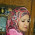## 27.11.14

### Excercise-17 : Function Progamming

Write a program using function to Sum of all Odd numbers from an Array. Use function prototype and parameter

1.#include
using namespace std;
int arraysum(int a[],int len);
main()
{
int len,j;
cout<<"the array size is:"<>len;
int a[len];
for(j=0;j>a[j];

arraysum(a,len);
}
int arraysum(int b[],int n)
{
int i,sum=0;
for(i=0;i<n;i++)
{
if(b[i]%2==1)
{
cout<<"Odd: "<<b[i]<<endl;
sum+=b[i];
}
}
cout<<sum;
}

1.2.Dear Sir, Please visit below blog:
http://itlearn24.blogspot.com/2014/11/write-program-using-function-to-sum-of.html

1.noted. its correct

3.#include
using namespace std;

void even_odd(int p)
{
if(p%2==0)
cout<<"Enter number is Even";
else
cout<<" nter number is Odd";
}

int main()
{
int n;
cout<<"Enter number to check Even or odd ";
cin>>p;
even_odd(p);
return 0;
}

4.#include
using namespace std;

int getsum( int j)
{
int a,sum=0;
cout<<"Enter "<>a[i];
}
for (int i=0;i>j;
cout<<endl<<"Sum of odd numbers is : "<<getsum(j)<<endl;
return 0;
}

5.This comment has been removed by the author.

6.#include
using namespace std;

class F
{
public:
void setnum(int p)
{
number = p;
}
protected: int number;
};
class findfact: public Factorial
{
public:
int fact=1;
void getfact()
{
for(int i = 1; i <= number; i++)
{
fact *= i;
}
cout<<"The Factorial value for the number is "<>p;
facts.setnum(n);
facts.getfact();
return 0;
}

7.#include
#include
using namespace std;

int character (string n)
{
int charnum=n.size();
for(char i=0; i<n.size(); i++)
{
if(n.at(i)== ' ')
{
charnum--;
}
if(n.at(i)== '.')
{
charnum--;
}
}
cout<<endl<<"Enter string has "<<charnum <<" Characters:"<<endl;
}

int main()
{
string n;
char i;
cout<<"Please type in a string: " ;
getline(cin,n);
character(n);
return 0;
}

8.This comment has been removed by the author.

9.#include
using namespace std;

int getsum( int b)
{
int a,sum=0;
cout<<"Enter "<>a[i];
}
for (int i=0;i>b;
cout<<endl<<"Sum of odd numbers is : "<<getsum(b)<<endl;
return 0;
}

10.This comment has been removed by the author.

11.Name : Sumsunnahar Nawa
ID : 201710090
Batch:54th

12.ID #201611036

13.MD Shipon Hossan
ID:201810009

14.ID # 201610288
Semester : Summer 2018
----------------------
Write any program to call a member function which will be cause of a different function to be executed depending on the types of object that invokes the function
---------------------
#include
#include //cmath file is included
using namespace std;

class areaCal{
public:
area(double x, double y){
calculate(x,y);
cout << "The area of the rectangle is : " << _a << endl;
}
area(double a, double b, double c){
calculate(a, b, c);
cout << "The area of the triangle is : " << _a << endl;
}
private:
double _a;

calculate(double x, double y){
_a = x*y;
}

calculate(double a, double b, double c){
double s;
s = (a+b+c)/2;
_a = sqrt(s*(s-a)*(s-b)*(s-c));
}

};

int main(){

double a, b, c;
cout << "Input first side of the triangle : ";
cin >> a;
cout << "Input first side of the triangle : ";
cin >> b;
cout << "Input first side of the triangle : ";
cin >> c;

areaCal tri;
tri.area(a,b,c);

cout << "-------------------------------" << endl;
cout << "-------------------------------" << endl;

double x, y;
cout << "Input length of the rectangle : ";
cin >> x;
cout << "Input width of the rectangle : ";
cin >> y;

areaCal rect;
rect.area(x,y);
}

Comment Here